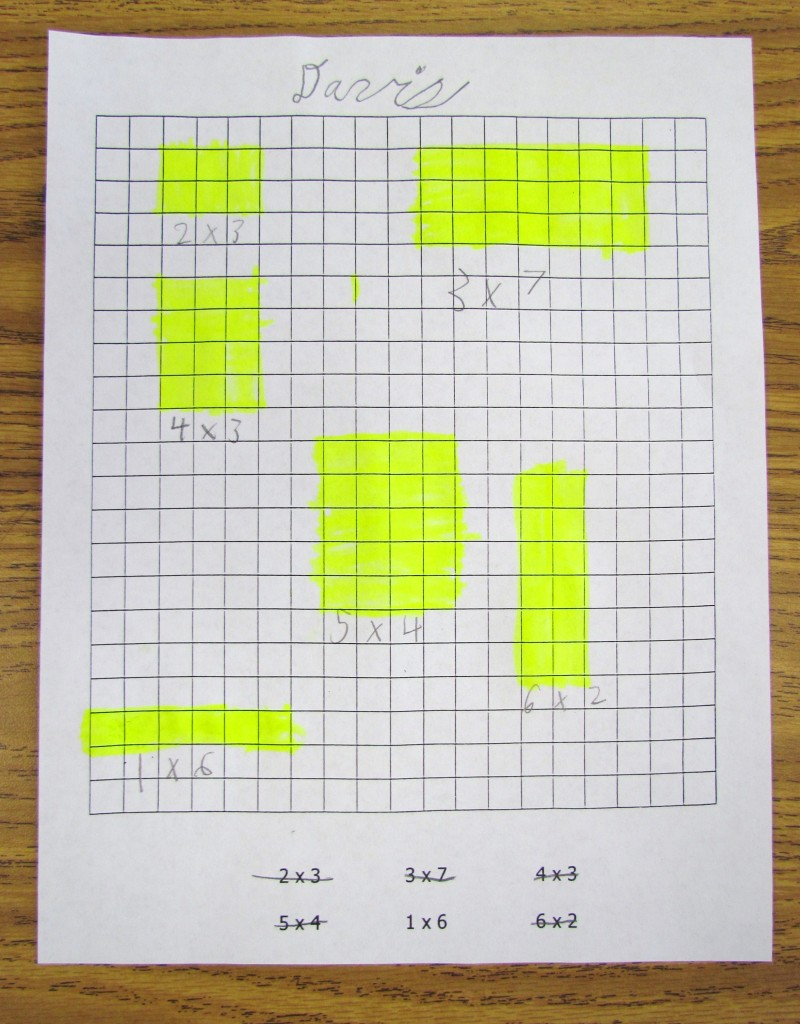March 27

# More Multiplication

We used a grid to demonstrate arrays. The equation 2×6 means two rows of six. We will connect this concept to area and perimeter later this week.Then we used the multiplication table to find products. This site would be an excellent way to practice multiplication facts this spring and through the summer.

Interactive multiplication table